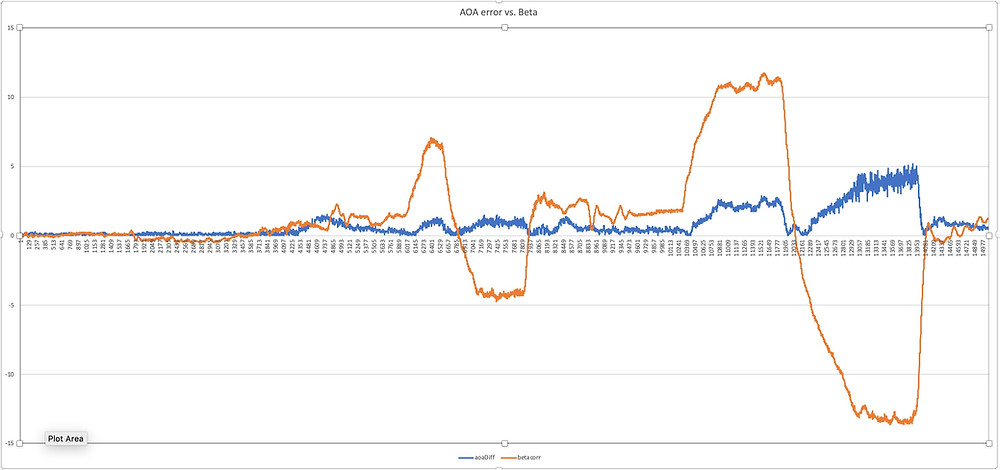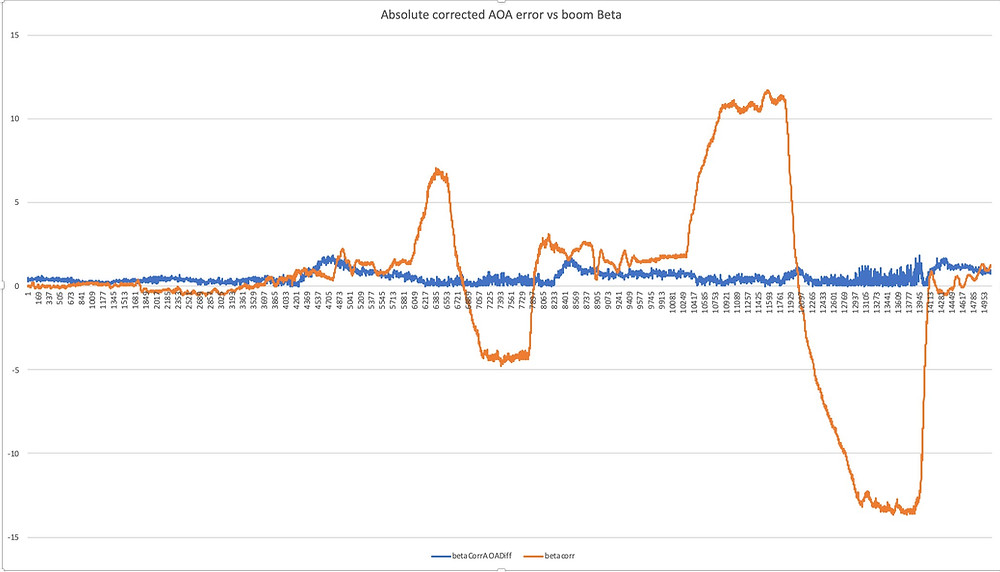Search

# Spring Cleaning

"OWS"

The F-15 had a nifty system that used tones (sound familiar?) when you approached the G-limit of the airplane. This system was called the Overload Warning System (or OWS). It knew if the the pull (G load) was symmetric or asymmetric. A symmetric pull is when the load is applied only in pitch, i.e., the stick is straight up and only moving aft. An asymmetric pull occurs when you pull AND roll at the same time. This asymmetric load is harder on the structure than a straight, symmetric pull. In fighters, we would teach folks to "unload, roll, set, pull." This meant first, you would reduce G load, then roll and only after the wings were set where you wanted them, would you pull straight aft. Often, we'd do this with two hands to ensure the stick was centered up, as it's easy to pull it to one side if you are aren't careful when flying with one hand. We called this a "zipper pull" since we wanted to keep the stick aligned with the front zipper on your flight suit. I still do a lot of two handed pulls in the RV-4 when I want to be double-dog sure that the stick is vertical.

Since the Gen 2 V3 has an IMU (inertial measurement unit), with 9 degrees of freedom, implementing G warning is pretty straight forward. The symmetric G limits are the published G limits of the airplane. We decided to just use the three categories spelled out in FAR 23: normal, utility and aerobatic. The pilot selects the category via the wifi interface. Since we are already using tones to define the aerodynamic limits of the envelope, we decided a simple "G Limit" call out was sufficient to remind the pilot when reaching limits, and there is no way to mix that up with the AOA tones.

We are still experimenting with how we're defining asymmetric maneuvering. For now, we are using a combination of G loading and roll rate. The rule of thumb we used in the military was 25% of maximum roll rate constitutes "asymmetric" maneuvering. But military airplanes (fighters in particular) are built to a higher structural margin than required for civilian airplanes (with the exception of some purpose designed aerobatic airplanes with structure optimized for snap maneuvering). In the military, asymmetric G limits are 20% less than symmetric, whereas for civilian airplanes, the certification requirement results in a 33% reduction. So, if we adjust the military rule of thumb; we end up with about 20% of maximum roll rate as constituting an asymmetric condition. The RV-4 has a maximum roll rate of about 150 deg per second...you know when you're there because there is a distinct bump felt through the stick when the down aileron stalls. If we apply our "civilian" rule of thumb to the typical RV, that means we ought to set the roll rate to 30 deg/second.

To be clear, our intent is to simply warn the pilot that the airplane is rolling approaching G limits. And realistically, unless the pilot has lost control of the velocity vector (where the airplane is pointing and how fast it's going), it's difficult in an airplane like the RV-4 to generate maximum G; so it's almost always below the asymmetric G limit (+4 in the RV-4, for example). Thus, I've currently got the software set to 15 degrees per second to assess the feature. We've also added a training and demonstration mode with an artificially low symmetric limit of 2.5 G's so pilots and flight instructors can experiment with "rolling G" warning well below the design limits of the airplane. In the future, we'll experiment with a vector sum OWS solution using some of the other axis measured by the IMU, if that is warranted.

Correcting for Beta

Besides alpha, the other greek letter of interest to our project is beta (sideslip or yaw angle). We know from Dr. Rogers original work, that at beta angles above 5-6 degrees, our simple coefficient of pressure sensors start accumulating more and more error as beta angle increases. As beta angle increases (say you are in a slipping base turn to final), the AOA solution begins to suffer error. Since we've got a calibrated beta reference on the test boom, we've been able to measure this error and have determined that it not only corrupts the alpha solution, it does so in an "optimistic" manner, i.e., the beta induced error results in lower than actual AOA. This is not good, since any spin/loss-of-control will likely involve alpha and beta excursions--which is a fancy way of saying stall and yaw. You can actually see this occurring if you pay close attention to the airspeed indicator during uncoordinated (e.g., in a slip) flight. The IAS will change slightly as the air flow angle across the pitot changes with yaw. And, you can hear some AOA tone "cut out" at high slip angles as well.

Figure 1 shows beta induced error. In this plot, left sideslip is expressed as a negative number, and right sideslip is expressed as a positive number. Power effects in the RV-4 allow me to generate more left yaw than right yaw. You can see in the plot, that at high beta angles, indicated AOA can be in error by 2 1/2 to almost 5 degrees. And error is greatest with left yaw, which unfortunately is the direction we typically do the most slipping in a standard traffic pattern.Figure 1. Beta-induced alpha error

Another way to depict the information in Figure 1 is an absolute value plot. Figure 2 is Figure 1 with beta expressed as absolute value:Figure 2: Absolute Value Plot, Beta-induced Alpha Error

Enter the HAL9000 Unit

Because a beta correction is likely going to be the result of a combination of vectors provided by the IMU, we wanted to see what's in the art of the doable with our inexpensive hardware. One analysis trick that we apply is the use of artificial intelligence. Training a neural network can make up for a glaring lack of math and physics skills, and give us a preview of an actual multi-vector solution. Neural networks can be a remarkably efficient way to solve a complex problem; but the draw back is that while we know what parameters we fed into the computer, we don't fully understand any resultant algorithm in a manner consistent with good (or at least current) engineering practice. But, as I said, our intent is simply to figure out if an inertial based solution is practical to provide a correction to computed alpha to compensate for side slip. And, turns out it is...Figure 3: HAL's IMU-derivived Beta Solution

You can see in Figure 3, HAL was able to figure out how to approximate beta angle using IMU inputs. While not perfect, it's certainly "good enough." What's more impressive is the HAL-generated algorithm to correct AOA error caused by yaw angle. Figure 4 shows a plot with AOA corrected for beta:Figure 4: AOA Corrected For Yaw Angle

Our objective is a true physics based IMU solution to apply to our AOA tone to ensure accuracy up to about 15 degrees of yaw. Remains to be seen if we'll be able to achieve that; but we're optimistic based on preliminary test!

Pitch-based Calibration

We've come up with an easier calibration technique: using pitch as a surrogate for alpha during level, unaccelerated flight. This is similar to the method used to calibrate the test boom on the airplane. Technically, it's possible to determine geometric angle of attack (the one we learned in pilot training: the difference between the relative wind and the chord line of the airplane) using this technique, but that requires two things: pitch adjusted to match the fuselage reference line of the airplane (this is the same "level" reference you use for weight and balance) and an accurate angle of incidence measurement. Being hand-built airplanes, exact incidence angle can sometimes be difficult to determine, and any builder error may deviate from the design incidence; so we've settled on using a "bore site" cue. Bore site occurs when pitch reference (the IMU in the V3 box, EFIS pitch, etc.) reads zero when the fuselage reference line is level. The resultant angle using this technique is neither absolute or geometric AOA using the standard definitions; however it's more than sufficient for generating an accurate aircraft curve. This logic will form the basis for our automatic calibration logic.

Again, to see what's in the art of the doable, we let HAL learn how to measure pitch using all of the V3 sensors:Figure 5. HAL's Pitch Solution

We used the pitch-based calibration solution for our Sling aircraft experiment, and have compared the results of Dave Rogers physics based approach with a pitch-derived curve in the instrumented RV-4 and found a nice correlation--it works just fine.

Log Curve Fit

For those that have been keeping up with our "hard tune" calibration efforts (which gives watching paint dry a run for its money on the 1-10 excitement scale...), you know we've been using a 2nd degree polynomial curve fit for the aircraft curves (i.e., that physics that allows us to accurately capture AOA within 1/2 degree or less throughout the envelope). If you think back to high-school math, and had to learn about graphing equations, you'll recall a 2nd order polynomial is a parabola. We'll that works pretty well up to stall angles, but at higher angles of attack the parabola starts to arc back over as parabolas are want to do, inducting some error in the AOA solution. Turns out a logarithmic curve fit is a better option for our software. This allows a pretty good capability to capture alpha angles post stall up to about 40 degrees or so as well as improving accuracy of the AOA solution at high (nearing stall) angles of attack. Switching to a log curve for the pressure solution and integrating the IMU will be the key to providing a LOC recovery display eventually.